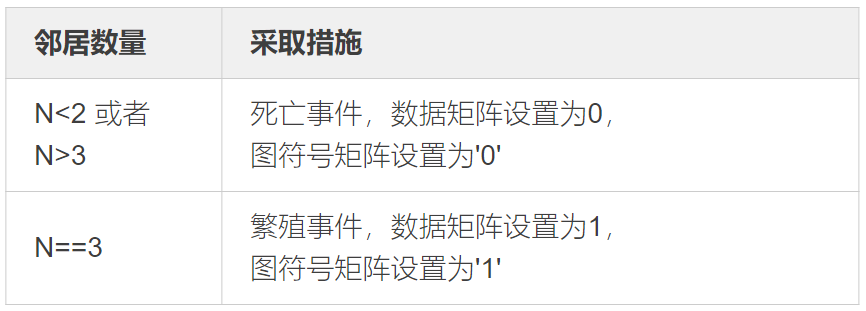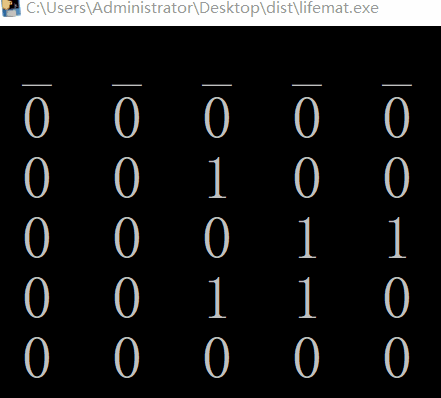# Python知识_面向对象三大特性_生命游戏_实例学习

XAMPP教程 285浏览

### 一、面向对象三大特性

##### （2）继承

”’
data : 2018.12.08
author : 老表
goal : 继承简单例子
”’

class allStr():
”’ 父类 ”’
all_words = ”欢迎关注简说Python!”
other_words = ”人生苦短，我选Python!”

def all_print(self):
”’ 打印其他的话 ”’
print(self.other_words)  # 调用自己的类变量

class myStr(allStr):
”’ 子类 ”’
def my_print(self):
”’ 打印我的话 ”’
print(allStr.all_words)  # 调用父类变量

# 初始化类对象
my_str = myStr()
# 调用类方法
my_str.my_print()
# 调用父类的方法
my_str.all_print()
# 企图外部直接修改父类变量
my_str.all_words = ”你好，我是老表，欢迎置顶公众号：简说Python!”
# 调用类方法
my_str.my_print()

”’
result :

”’

##### （3）多态

###### 重写

“”"
author : 老表
data : 2018.12.09
goal : 多态实例
“”"

class AllStr:
“”" 父类 ”"”
all_words = ”你好，简说Python!”
my_words = ”欢迎关注：简说Python!”

def print_str(self):
print(self.all_words)

class MyStr(AllStr):
“”" 子类 ”"”
def print_str(self):
“”" 重写父类的方法 ”"”
print(self.my_words)

def print_me(self):
“”" 属于自己的方法 ”"”
print(“人生苦短，快学Python!”)

def print_me(self, your_words):
“”" 重载自己类里的方法 ”"”
print(your_words)

# 初始化一个对象
my_str = MyStr()
# 调用重写父类的方法
my_str.print_str()
# 调用自己特有的方法
# my_str.print_me() 重载后，之前的方法不可调用
# 调用子类自己重载的方法
your_words = ”我置顶了 简说Python!”
my_str.print_me(your_words)
”’
result :

”’

### 二、生命游戏

#### 基本规律#### (1)Matrix2D类代码实现

“”"

“”"

class Matrix2D:
“”" 通用的二维矩阵类 ”"”

def __init__(self, rows, cols):
“”" 初始化矩阵row行，col列 ”"”
self.grid = [*cols for _ in range(rows)]
self.rows = rows
self.cols = cols

def get_cell(self, r, c):
“”" 获取单元格(r,c)的值 ”"”
return self.grid[r][c]

def set_cell(self, n, **args):
“”" 设置某个位置的值 ”"”
for r, c in args:
self.grid[r][c] = n

def inc_cells(self, **args):
“”" 将任意的单元格 ＋1 ”"”
for r, c in args:
self.grid[r][c] += 1

def set_all_cells(self, n=0):
“”" 将所有单元格值都设置为 n ”"”
for i in range(self.rows):
for j in range(self.cols):
self.grid[i][j] = n

##### (2)主函数

“”"

“”"

from lifemat import Matrix2D

rows = 5
cols = 5
# 存储图符号的二维数组
life_mat = Matrix2D(rows, cols)
# 存储具体数据的二维数组
nc_mat = Matrix2D(rows, cols)
# 初始化
life_mat.set_cells(1, (1, 3), (2, 1), (2, 3), (3, 2), (3, 3))
# 创建边界字符串
border_str = ’ _ ’ * cols

def get_mat_str(a_mat):
“”" 处理打印字符串 ”"”
disp_str = ”
for i in range(rows):
lst = [get_chr(a_mat, i, j) for j in range(cols)]
disp_str += ”.join(lst) + ’\n’
return disp_str

def get_chr(a_mat, r, c):
“”" 设置图符号 ”"”
return ’ 1 ’ if a_mat.get_cell(r, c) > 0 else ’ 0 ’

def do_generation():
“”" 打印当前状态并生成下个状态 ”"”
# 打印当前生命矩阵状态
print(border_str + ’\n’ + get_mat_str(life_mat))
# 把数据全部置0
nc_mat.set_all_cells(0)

# 根据图符号矩阵life_mat来给nc_mat赋值

for i in range(rows):
for j in range(cols):
if life_mat.get_cell(i, j):
# 环绕图像，使有限的二维数组变成没有边界的生命游戏
im = (i - 1) % rows
ip = (i + 1) % rows   # 当前行号-/+ 1
jm = (j - 1) % cols
jp = (j + 1) % cols   # 当前列号-/+ 1
# 设置数据量为 1 ，表示有活细胞
nc_mat.inc_cells((im, jm), (im, j), (im, jp), (i, jm),
(i, jp), (ip, jm), (ip, j), (ip, jp))
# 根据邻居数量矩阵按规则生成下一代
for i in range(rows):
for j in range(cols):
n = nc_mat.get_cell(i, j)
if n < 2 or n > 3:      # 死亡现象
life_mat.set_cells(0, (i, j))
elif n == 3:            # 繁殖现象
life_mat.set_cells(1, (i, j))

import time
n = 100
for i in range(n):
# 循环调用迭代
do_generation()
# 设置时间间隔
time.sleep(1)

#### 运行效果《零压力学Python》里说，学习新编程语言的时候，如果能使用它编写出生命游戏，就说明掌握了这门语言，编完后，我觉得是有很大道理的，不说所有，能弄懂这个程序，至少可以说明你的基础过关了。

### 三、装饰器

#### 实例学习

##### 1.最简单的例子

def my_decorator(f):
“”" 装饰器，将一个函数作为参数传递进来，进行包装，然后返回 ”"”
def wrapper():
print(“I am doing extra stuff.”)
f()
print(“Doing more extra stuff.”)
return wrapper

def hello():
print(“简说Python 你好！”)

new_hello = my_decorator(hello)
new_hello()
”’
result:
I am doing extra stuff.

Doing more extra stuff.
”’

##### 2.中等简单

def my_decorator(f):
“”" 装饰器，将一个函数作为参数传递进来，进行包装，然后返回 ”"”
def wrapper():
print(“I am doing extra stuff.”)
f()
print(“Doing more extra stuff.”)
return wrapper

”’

def hello():
print(“Hi，简说Python!”)
hello = my_decorator(hello)
”’
@my_decorator
def hello():
print(“Hi，简说Python!”)

hello()

”’
result:
I am doing extra stuff.
Hi，简说Python!
Doing more extra stuff.
”’

##### 3.复杂案例

from time import time

def diagnostics(f):
def wrapper(*args, **kwargs):
“”" 这个包装函数带参数，
*args处理多个参数，
**kwargs可以处理具名参数 ”"”
print(“Executed”, f.__name__, ”at”, time())
value = f(*args, **kwargs)
print(“Exited”, f.__name__, ”at”, time())
print(“Arguments:”,args)
print(“Value returned:”, value, ”\n”)
return value
return wrapper

@diagnostics
def print_nums():
“”" 不带参数，没有返回值 ”"”
for i in range(4):
print(i, end=”\t”)

@diagnostics
“”" 带参数，有返回值 ”"”
return a+b

# 调用经过装饰器装饰过的函数
print_nums()
print(“-”*50)
print(“-”*50)

”’
result:
Executed print_nums at 1544353643.8159559
0   1   2   3   Exited print_nums at 1544353643.8159559
Arguments: ()
Value returned: None

————————————————–